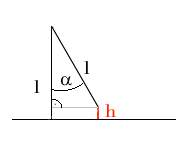A bag filled with sand, weighing m2 = 2 kg, is hanging freely, connected to a nail attached to the wall with a massive rope of a length of l = 0.1 m. At first it is not moving. Now someone throws a tennis ball weighing m1 = 0.1 kg with an average speed of v = 21 m/s directly to its center of gravity. The bag thus accelerates while the ball decelerates until both of them are moving at the same speed v'. Assume that the acceleration of gravity g = 10 m/s^2. What's the angle alpha by which the rope has rotated (see sketch)?